Courses

# Minimum Spanning Trees MCQ - 1

## 20 Questions MCQ Test RRB JE for Computer Science Engineering | Minimum Spanning Trees MCQ - 1

Description
This mock test of Minimum Spanning Trees MCQ - 1 for Computer Science Engineering (CSE) helps you for every Computer Science Engineering (CSE) entrance exam. This contains 20 Multiple Choice Questions for Computer Science Engineering (CSE) Minimum Spanning Trees MCQ - 1 (mcq) to study with solutions a complete question bank. The solved questions answers in this Minimum Spanning Trees MCQ - 1 quiz give you a good mix of easy questions and tough questions. Computer Science Engineering (CSE) students definitely take this Minimum Spanning Trees MCQ - 1 exercise for a better result in the exam. You can find other Minimum Spanning Trees MCQ - 1 extra questions, long questions & short questions for Computer Science Engineering (CSE) on EduRev as well by searching above.
QUESTION: 1

Solution:
QUESTION: 2

Solution:
QUESTION: 3

### Consider a complete undirected graph with vertex set {0, 1, 2, 3, 4}. Entry Wij in the matrix W below is the weight of the edge {i, j}. What is the minimum possible weight of a spanning tree T in this graph such that vertex 0 is a leaf node in the tree T?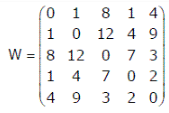Solution:

To get the minimum spanning tree with vertex 0 as leaf, first remove 0th row and 0th column and then get the minimum spanning tree (MST) of the remaining graph. Once we have MST of the remaining graph, connect the MST to vertex 0 with the edge with minimum weight (we have two options as there are two 1s in 0th row).

QUESTION: 4

In the graph given in above question question, what is the minimum possible weight of a path P from vertex 1 to vertex 2 in this graph such that P contains at most 3 edges?

Solution:

Path: 1 -> 0 -> 4 -> 2 Weight: 1 + 4 + 3

QUESTION: 5

An undirected graph G has n nodes. Its adjacency matrix is given by an n × n square matrix whose
(i) diagonal elements are 0‘s and
(ii) non-diagonal elements are 1‘s.
which one of the following is TRUE?

Solution:
QUESTION: 6

Consider the following graph: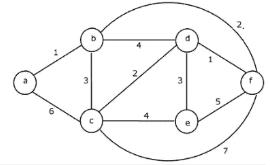Q. Which one of the following cannot be the sequence of edges added, in that order, to a minimum spanning tree using Kruskal’s algorithm?

Solution:

The edge (d-e) cannot be considered before (d-c) in Kruskal's minimum spanning tree algorithm because Kruskal’s algorithm picks the edge with minimum weight from the current set of edges at each step.

QUESTION: 7

Let G be an undirected connected graph with distinct edge weight. Let emax be the edge with maximum weight and emin the edge with minimum weight. Which of the following statements is false? (GATE CS 2000)

Solution:

(a) and (b) are always true. (c) is false because (b) is true. (d) is true because all edge weights are distinct for G.

QUESTION: 8

Consider a weighted complete graph G on the vertex set {v1,v2 ,v} such that the weight of the edge (v,,v) is 2|i-j|. The weight of a minimum spanning tree of G is:

Solution:

Minimum spanning tree of such a graph is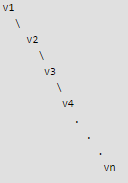Weight of the minimum spanning tree = 2|2 - 1| + 2|3 - 2| + 2|4 - 3| + 2|5 - 4| .... + 2| n - (n-1) | = 2n - 2

QUESTION: 9

Let G be a weighted graph with edge weights greater than one and G'be the graph constructed by squaring the weights of edges in G. Let T and T' be the minimum spanning trees of G and G', respectively, with total weights t and t'. Which of the following statements is TRUE?

Solution:

Squaring the weights of the edges in a weighted graph will not change the minimum spanning tree. Assume the opposite to obtain a contradiction. If the minimum spanning tree changes then at least one edge from the old graph G in the old minimum spanning tree T must be replaced by a new edge in tree T' from the graph G' with squared edge weights. The new edge from G' must have a lower weight than the edge from G. This implies that there exists some weights C1 and C2 such that C1 < C2 and C12 >= C22. This is a contradiction. Sums of squares of two or more numbers is always smaller than square of sum. Example: 2^2 + 2^2 < (4)^2 But there is one counter example when the graph has only one edge. In that case, the two values are same.

QUESTION: 10

Consider the following graph: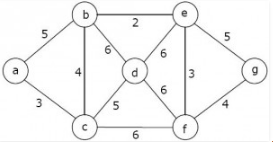Q. Which one of the following is NOT the sequence of edges added to the minimum spanning tree using Kruskal's algorithm?

Solution:

In the sequence (b, e) (e, f) (b, c) (a, c) (f, g) (c, d) given option D, the edge (a, c) of weight 4 comes after (b, c) of weight 3. In Kruskal's Minimum Spanning Tree Algorithm, we first sort all edges, then consider edges in sorted order, so a higher weight edge cannot come before a lower weight edge.

QUESTION: 11

The number of distinct minimum spanning trees for the weighted graph below is ____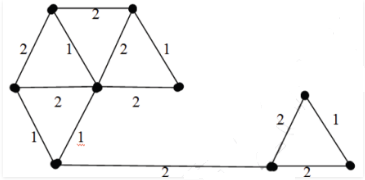Solution:

Below diagram shows a minimum spanning tree. Highlighted (in green) are the edges picked to make the MST.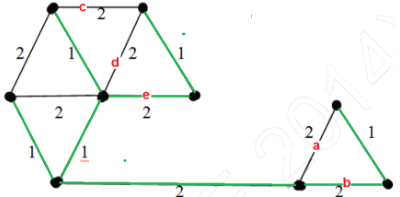In the right side of MST, we could either pick edge ‘a’ or ‘b’. In the left side, we could either pick ‘c’ or ‘d’ or ‘e’ in MST. There are 2 options for one edge to be picked and 3 options for another edge to be picked. Therefore, total 2*3 possible MSTs.

QUESTION: 12

Let s and t be two vertices in a undirected graph G + (V, E) having distinct positive edge weights. Let [X, Y] be a partition of V such that s ∈ X and t ∈ Y. Consider the edge e having the minimum weight amongst all those edges that have one vertex in X and one vertex in Y The edge e must definitely belong to:

Solution:

The minimum weight edge on any s-t cut is always part of MST. This is called Cut Property. This is the idea used in Prim's algorithm. The minimum weight cut edge is always a minimum spanning tree edge. Why B (the weighted shortest path from s to t) is not an answer? See below example, edge 4 (lightest in highlighted red cut from s to t) is not part of shortest path.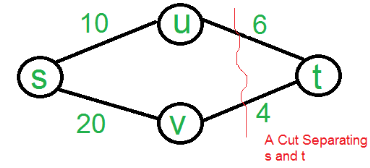QUESTION: 13

What is the weight of a minimum spanning tree of the following graph ?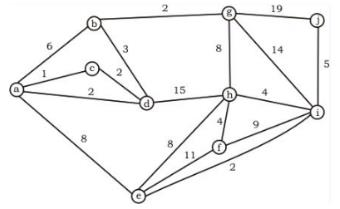Solution:

(a,c), (a,d), (d,b), (b,g), (g,h), (h,f), (h,i), (i,j), (i,e) = 31   Background required - Minimum Spanning Tree (Prims / Kruskal) In these type of questions, always go for kruskal’s algorithm to find out the the minimum spanning tree as it is easy and there are less chances of doing silly mistakes. Algorithm: Always pick the minimum edge weight and try to add to current forest (Collection of Trees) if no cycle is formed else discard. As soon as u have added n-1 edges to the forest, stop and you have got your minimum spanning tree. See the below image for construction of MST of this question.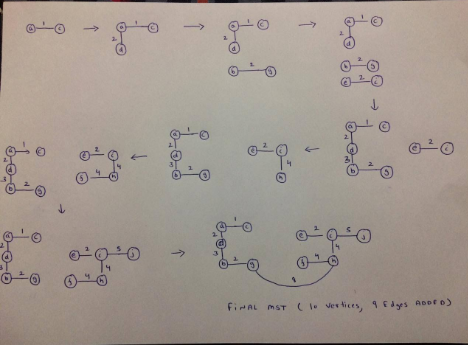Weight of minimum spanning tree = Sum of all the edges in Minimum Spanning tree = 31

QUESTION: 14

Consider an undirected unweighted graph G. Let a breadth-first traversal of G be done starting from a node r. Let d(r, u) and d(r, v) be the lengths of the shortest paths from r to u and v respectively in G. If u is visited before v during the breadth-first traversal, which of the following statements is correct?

Solution:
QUESTION: 15

The graph shown below 8 edges with distinct integer edge weights. The minimum spanning tree (MST) is of weight 36 and contains the edges: {(A, C), (B, C), (B, E), (E, F), (D, F)}. The edge weights of only those edges which are in the MST are given in the figure shown below. The minimum possible sum of weights of all 8 edges of this graph is ______________.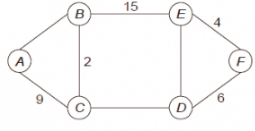Solution:

In every cycle, the weight of an edge that is not part of MST must by greater than or equal to weights of other edges which are part of MST. Since all edge weights are distinct, the weight must be greater. So the minimum possible weight of ED is 7, minimum possible weight of CD is 16 and minimum possible weight of AB is 10. Therefore minimum possible sum of weights is 69.

QUESTION: 16

Let G be connected undirected graph of 100 vertices and 300 edges. The weight of a minimum spanning tree of G is 500. When the weight of each edge of G is increased by five, the weight of a minimum spanning tree becomes ________.

Solution:

Since there are 100 vertices, there must be 99 edges in Minimum Spanning Tree (MST). When weight of every edge is increased by 5, the increment in weight of MST is = 99 * 5 = 495 So new weight of MST is 500 + 495 which is 995

QUESTION: 17

Let G be a weighted connected undirected graph with distinct positive edge weights. If every edge weight is increased by the same value, then which of the following statements is/are TRUE?

P: Minimum spanning tree of G does not change
Q: Shortest path between any pair of vertices does not change

Solution:

The shortest path may change. The reason is, there may be different number of edges in different paths from s to t. For example, let shortest path be of weight 15 and has 5 edges. Let there be another path with 2 edges and total weight 25. The weight of the shortest path is increased by 5*10 and becomes 15 + 50. Weight of the other path is increased by 2*10 and becomes 25 + 20. So the shortest path changes to the other path with weight as 45. The Minimum Spanning Tree doesn't change. Remember the Kruskal's algorithm where we sort the edges first. IF we increase all weights, then order of edges won't change.

QUESTION: 18

Let G be a complete undirected graph on 4 vertices, having 6 edges with weights being 1, 2, 3, 4, 5, and 6. The maximum possible weight that a minimum weight spanning tree of G can have is.

Solution:

One graph that has maximum possible weight of spanning tree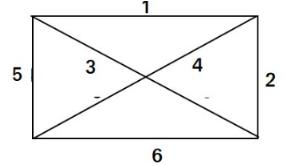QUESTION: 19

G = (V, E) is an undirected simple graph in which each edge has a distinct weight, and e is a particular edge of G. Which of the following statements about the minimum spanning trees (MSTs) of G is/are TRUE

I. If e is the lightest edge of some cycle in G, then every MST of G includes e
II. If e is the heaviest edge of some cycle in G, then every MST of G excludes e

Solution:

I is NOT true. Let G=(V, E) be a rectangular graph where V = {a, b, c, d} and E = {ab, bc, cd, da, ac}. Let the edges have weights: ab = 1, bc = 2, cd = 4, da = 5, ac = 3. Then, clearly, ac is the lightest edge of the cycle cdac, however, the MST abcd with cost 7 (= ab + bc + cd) does not include it. Let the edges have weights: ab = 6, bc - 7, cd = 4, da = 5, ac = 3. Then, again, ac is the lightest edge of the cycle cdac, and, the MST bacd with cost 13 (= ba + ac + cd) includes it. So, the MSTs of G may or may not include the lightest edge.

II is true Let the heavies edge be e. Suppose the minimum spanning tree which contains e. If we add one more edge to the spanning tree we will create a cycle. Suppose we add edge e' to the spanning tree which generated cycle C. We can reduce the cost of the minimum spanning tree if we choose an edge other than e from C for removal which implies that e must not be in minimum spanning tree and we get a contradiction.

QUESTION: 20

What is the largest integer m such that every simple connected graph with n vertices and n edges contains at least m different spanning trees?

Solution:

A graph is connected iff all nodes can be traversed from each node. For a graph with n nodes, there will be n-1 minimum number of edges.
Given that there are n edges, that means a cycle is there in the graph.
The simplex graph with these conditions may be: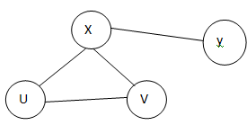Now we can make a different spanning tree by removing one edge from the cycle, one at a time.
Minimum cycle length can be 3, So, there must be atleast 3 spanning trees in any such Graph.